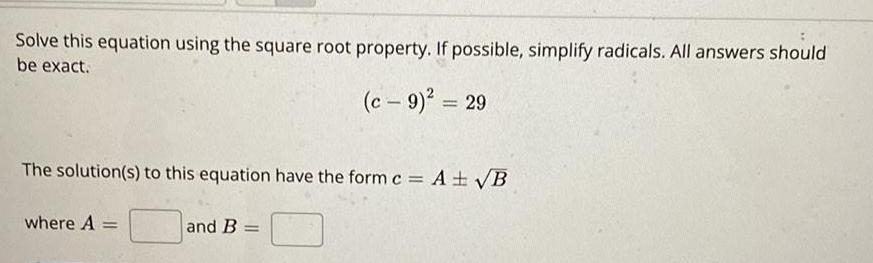Question:

# Solve this equation using the square root property. If

Last updated: 8/14/2022Solve this equation using the square root property. If possible, simplify radicals. All answers should be exact. (c - 9)² = 29 The solution(s) to this equation have the form c = A ± √B